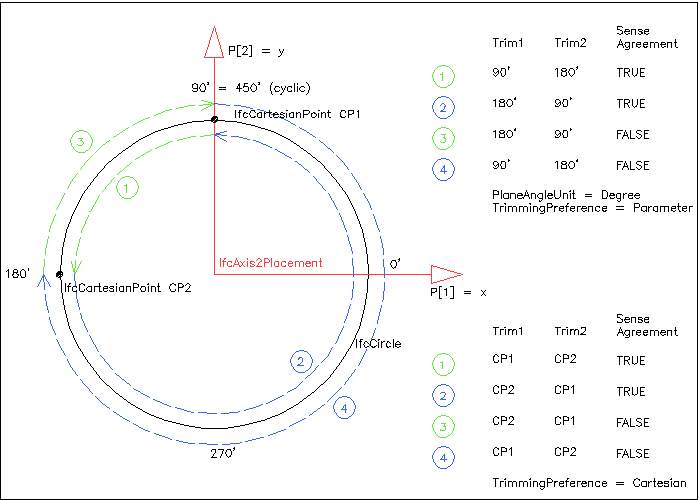# 8.9.3.75 IfcTrimmedCurve

## 8.9.3.75.1 Semantic definition

An IfcTrimmedCurve is a bounded curve that is trimmed at both ends. The trimming points may be provided by a Cartesian point or by a parameter value, based on the parameterization of the BasisCurve. The SenseAgreement attribute indicates whether the direction of the IfcTrimmedCurve agrees with or is opposed to the direction of the BasisCurve.Figure 8.9.3.75.A shows the four arcs (dashed blue and green lines with arrow showing different orientations) that can be defined by the same BasisCurve (of type IfcCircle) and the same trimming points (given by Cartesian points and parameter values) by using different assignments to Trim1 and Trim2 and SenseAgreement.

At least one of these shall be specified at each end of the curve. The SenseAgreement makes it possible to unambiguously define any segment of a closed curve such as a circle. The combinations of sense and ordered end points make it possible to define four distinct directed segments connecting two different points on a circle or other closed curve. For this purpose cyclic properties of the parameter range are assumed; for example, 370 degrees is equivalent to 10 degrees.

The IfcTrimmedCurve has a parameterization which is inherited from the particular basis curve reference. More precisely the parameter s of the trimmed curve is derived from the parameter of the basis curve as follows:

• if SenseAgreement is TRUE: s = t - t~1~
• if SenseAgreement is FALSE: s = t~2~ - t

In the above equations t~1~ is the value given by Trim1 or the parameter value corresponding to point 1 and t~2~ is the value given by Trim2 or the parameter value corresponding to point 2. The resultant IfcTrimmedCurve has a parameter ranging from 0 at the first trimming point to |t~2~ - t~1~| at the second trimming point.

Informal Propositions:

1. Where both the parameter value and the Cartesian point exist for Trim1 and Trim2 they shall be consistent. (i.e., the BasisCurve evaluated at the parameter value shall coincide with the specified point).
2. When a Cartesian point is specified by Trim1 or by Trim2 it shall lie on the BasisCurve.
3. Except the case of a closed BasisCurve where both parameter 1 and parameter 2 exist, they shall be consistent with the sense flag, i.e., (sense = parameter 1 < parameter 2). Or, for every open curve where both parameter 1 and parameter 2 exist, they shall be consistent with the SenseAgreement, i.e., SenseAgreement = (parameter 1 < parameter 2).
4. If both parameter 1 and parameter 2 exist, then parameter 1 <> parameter 2. For a closed base curve, e.g. IfcCircle or IfcEllipse, this also applies to the cyclic properties, as 360' is equal to 0', parameter 1 = 360' and parameter 2 = 0' are treated as being equal and therefore violating this proposition.
5. When a parameter value is specified by Trim1 or Trim2 it shall lie within the parametric range of the BasisCurve.

## 8.9.3.75.5 Formal representation

ENTITY IfcTrimmedCurve
SUBTYPE OF (IfcBoundedCurve);
BasisCurve : IfcCurve;
Trim1 : SET [1:2] OF IfcTrimmingSelect;
Trim2 : SET [1:2] OF IfcTrimmingSelect;
SenseAgreement : IfcBoolean;
MasterRepresentation : IfcTrimmingPreference;
WHERE
NoTrimOfBoundedCurves : NOT('IFC4X3_DEV_9f90c1f0.IFCBOUNDEDCURVE' IN TYPEOF(BasisCurve));
Trim1ValuesConsistent : (HIINDEX(Trim1) = 1) OR (TYPEOF(Trim1) <> TYPEOF(Trim1));
Trim2ValuesConsistent : (HIINDEX(Trim2) = 1) OR (TYPEOF(Trim2) <> TYPEOF(Trim2));
END_ENTITY;